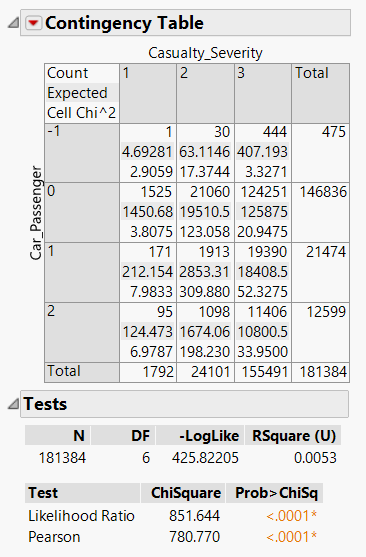Our World Statistics Day conversations have been a great reminder of how much statistics can inform our lives. Do you have an example of how statistics has made a difference in your life? Share your story with the Community!
Choose Language Hide Translation Bar
Highlighted

## Help me make sense of the significance in Chi-Square Analyses results

Hey all,

Here I have run the presense of a car passenger (1 = front seat, 2=back seat) against casualty severity (1 = fatal, 2 = serious, 3 = slight).

I am having trouble understand the results. I understand that the expected count and the actual count is what I'm interested in, but how do I detect whether the difference between those two counts is significant.

4 REPLIES 4
Highlighted

## Re: Help me make sense of the significance in Chi-Square Analyses resultsHighlighted

## Re: Help me make sense of the significance in Chi-Square Analyses results

If there is no association, then the marginal distribution holds regardless of the level of the predictor variable. This quantity is provided by the column totals (count) at the bottom of the contingency table. The Expected value is based on the marginal distribution. So the Expected value for the first cell for Severity=1 and Car Passenger=-1 is 475*(1792/181384) and so on. The observed count is compared to the value expected if there is no association using a chi square distance: (Count-Expected)^2 / Expected. The chi square for the first cell is (1-4.69281)^2 / 4.69281 = 2.9059. The square root of the cell chi square is the Pearson residual and gives you an idea of the levels that are most important to the alternative hypothesis. Finally, you add all the cell statistics to obtain the sample chi square (labelled as Pearson in the bottom table). The sample statistic follows a chi square distribution under the null hypothesis and a large sample. The expected value is the degrees of freedom = (n row - 1)(n col - 1) = (4-1)(3-1) = 6. Your sample statistic 780.77 is much larger than 6 indicating strong evidence against the null hypothesis.

Your sample is very large so it is possible to detect weak associations. While the evidence strongly suggests the alternative hypothesis (there is an association), the R square value 0.0053 indicates that this association does not provide much predictive information. The odds ratio would indicate the strength of the association.

Learn it once, use it forever!
Highlighted

## Re: Help me make sense of the significance in Chi-Square Analyses results

Mr.Bailey,

"Your sample is very large so it is possible to detect weak associations. While the evidence strongly suggests the alternative hypothesis (there is an association), the R square value 0.0053 indicates that this association does not provide much predictive information. The odds ratio would indicate the strength of the association."

Ok, awesome. The odds ratio you mention, is that the ratio between the expected and the observed?

Highlighted

## Re: Help me make sense of the significance in Chi-Square Analyses results

No, an odds ratio represents the strength of the association, not the significance (chi square sample statistic), so it is the ratio of the odds of an outcome for different levels of the predictor or explanatory variable. For example, you might ask what is the odds ratio for Severity = 3 when Passenger = 0 versus Passenger = 2.

You need to use Analyze > Fit Model in order Use Ordinal Logistic platform to fit the logistic regression model. Then save the probability formulas that can then be used to compute the odds and the desired odds ratios.

Learn it once, use it forever!
Article Labels

There are no labels assigned to this post.